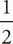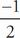# SAT Math Multiple Choice Question 104: Answer and Explanation

### Test Information

Question: 104

14. The function g is defined by g(x) = 2x2 - dx - 6, where d is a constant. If one of the zeros of g is 6, what is the value of the other zero of g ?

• A. 2
• B.• C. -• D. -2

C The zero of g is the value of the variable, in this case x, when the equation is set to 0. This is also called the root or solution of an equation. Set the equation to 0 to get 0 = 2x2 - dx - 6. Plug 6 in for x to get 0 = 2(62) - d(6) - 6. Simplify the equation to get 0 = 72 - 6d - 6, or 0 = 66 - 6d. Solve for d to get -66 = -6d, so 11 = d. Plug 11 in for d and set the quadratic to 0 to get 0 = 2x2 - 11x - 6. Factor the equation to get 0 = (x - 6)(2x + 1). The other zero of the equation is when 2x + 1 = 0. Solve for x to get 2x = -1, or x =. The correct answer is (C).